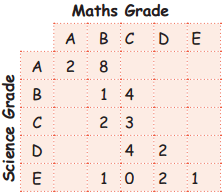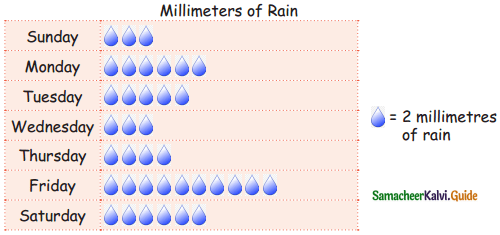Students can download 5th Maths Term 1 Chapter 6 Information Processing Ex 6.3 Questions and Answers, Notes, Samacheer Kalvi 5th Maths Guide Pdf helps you to revise the complete Tamilnadu State Board New Syllabus, helps students complete homework assignments and to score high marks in board exams.

## Tamilnadu Samacheer Kalvi 5th Maths Solutions Term 1 Chapter 6 Information Processing Ex 6.3

Question 1.
The bar chart represents the number of students using different modes of transport. Observe and answer the following questions.a. Which mode of transport is mostly used by the students?
Bicycle.

b. How many students come to school by walking?
40 students.

c. Which mode of transport is used the least?
car.

d. How many students come to school by Bus?
40 students.Question 2.
The following information shows the grades of science and maths of 30 students of class 5.a. How many students got equal grades in both subjects.
Two

b. How many students got a higher grade in science than in maths?
Eight

c. Which was the most common grade in science?
c.Question 3.
Mr. binesh collected the information about the rainfall of a particular city in a week from the newspaper and recorded his information in the pictograph.a. On which day, the rain was most?
Friday (20 mm).

b. On which day, the rain was least?
Sunday & Wednesday (6 mm).

c. How much rain was there on Sunday?
6 mm.

d. How much rain was there on Monday?
12 mm.

e. Find total rainfall of the city in that week?
74 mm.Question 4.
NeeIa, Mala, Kola and Balo were neighbours. The following dato shows the number of fish in each of their fish tank, brow pictogroph to represent the data and Answer the questions.a. How many fishes did bala have? ________